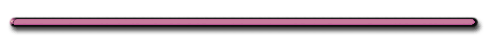HomeWhat would happen if this bottle were dropped?

Newton's Masterpiece!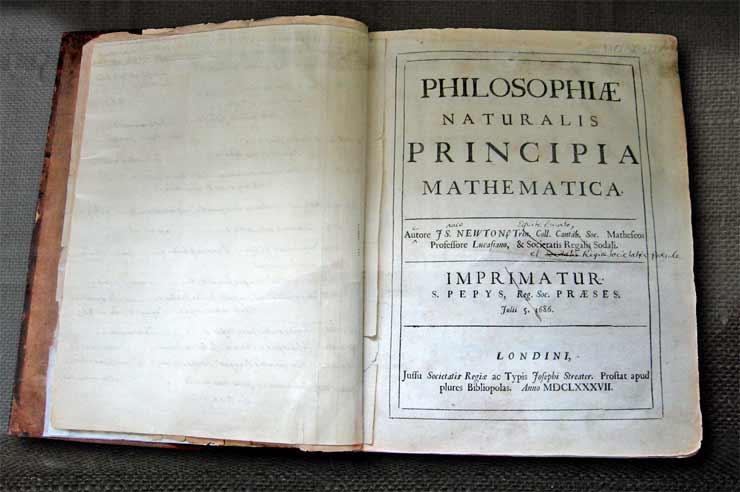Sir Isaac Newton's own first edition copy of his Philosophiae Naturalis Principia Mathematica
with his handwritten corrections for the second edition.

The first edition was published under the imprint of Samuel Pepys who was president of the Royal Society. By the time of the second edition, Newton himself had become president of the Royal Society, as noted in his corrections.

The book can be seen in the Wren Library of Trinity College, Cambridge.

 Photograph © Andrew Dunn, 5 November 2004. Website: http://www.andrewdunnphoto.com/

Review

When  is the magnitude of the acceleration constant, increasing, decreasing or zero?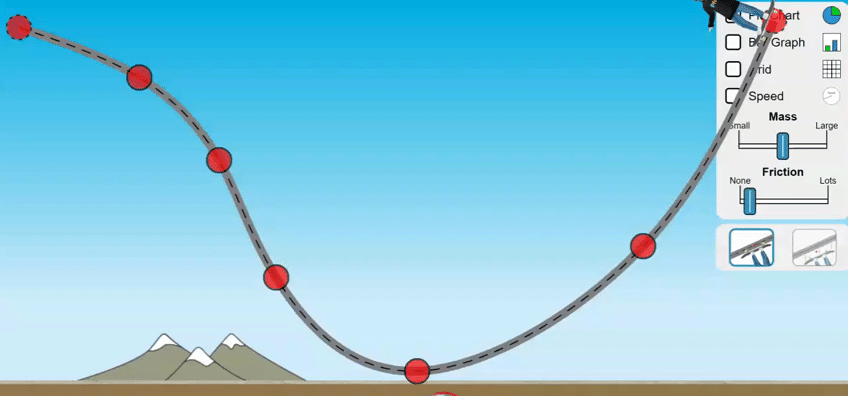4. Standard Equation

 Fnet = ma (N) (Kg)(m/s2)

Newton - force needed to give a 1 kg of mass an acceleration of 1 m/s2Derived unit - A unit that comes from the combination of other units

N = Kgm/s2

Fundamental unit
(opposite) m, s, KgForce Questions

 Ex 1) What is the net force necessary to give a 3.0 kg mass, initially at rest, an acceleration of 5.0 m/s2?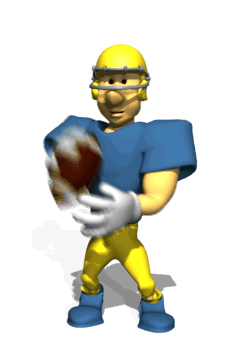Ex 1) What is the force necessary to give a 3.0 kg mass, initially at rest, an acceleration of 5.0 m/s2?

F = ma(3.0 kg)(5.0 m/s2)

= 15 N

or

15 kgm/s2Ex 2) A net force of 30. nt accelerates an object by 15. m/s2. Find the objects mass..

Ex 2) A force of 30. nt accelerates an object by 15. m/s2.

Find the objects mass.

F = ma

30. N = m (15. m/s2)

m = 2.0 kgEx 3) An unbalanced force of 100. nt acts on a 50. kg mass for 5.0 seconds.    Find the acceleration of the mass.

Ex 3) An unbalanced force of 100. nt acts on a 50. kg mass for 5.0 seconds.

Find the acceleration of the mass

Fnet = ma

100. nt = (50. kg) a

a = 2.0 m/s2Ex 4) A 10. kg cart moving with a velocity of 2.0 m/s is brought to a stop in 2.0 sec.  Find magnitude of the average force used to stop the cart.

Ex 4) A 10. kg cart moving with a velocity of 2.0 m/s is brought to a stop in 2.0 sec

Find magnitude of the average force used to the stop cart.

m = 10. kg

t = 2.0 sec

Vi = 2.0 m/s

Vf = 0 m/s

(stop)

F = ?

F = ma

a = ?

 a = Vf  - Vi = 0 - 2.0 m/s t 2.0 sec

a = -1.0 m/s2

Fnet = ma

= (10. kg)(-1.0 m/s2)

= -10. N

or

-10. kgm/s2Ex 5) The object below has a mass of 5 kg. Find its acceleration.Fnet = ma

40 N East = (5 kg)a

a = 8 m/s2 East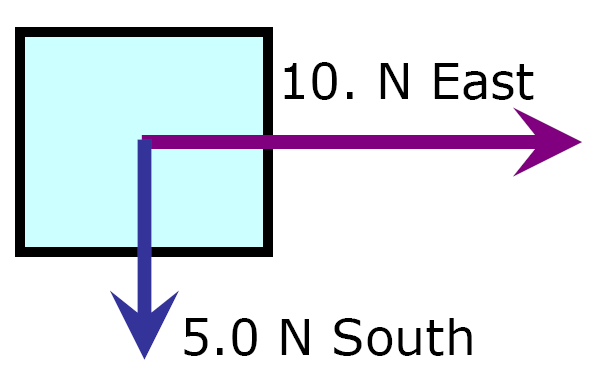Ex 6) The block above has an acceleration of 22. m/s2. What is the mass of the block?

5N2 + 10N2 = R2

Fnet = ma

Fnet=11 N=m(22 m/s2)

m = .50 kg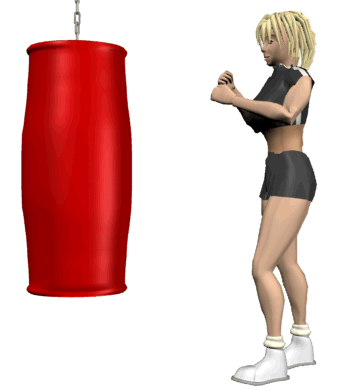Ex 7) If the magnitude of the block’s acceleration is 2.0 m/s2, what is the mass of the block?

Fnet = ma

10 N Left = m(2 m/s2)

m = 5 kg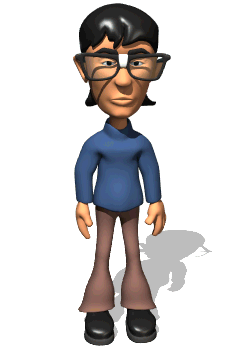What will the balloon do when the cart is pushed to the left?

(Click on image)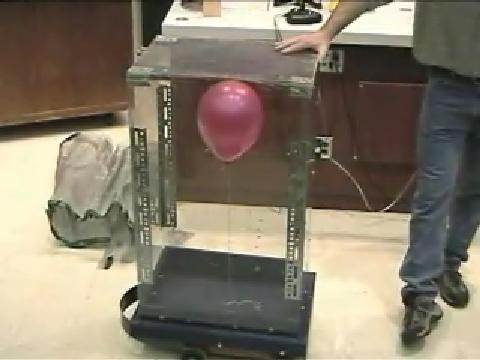The air in the container tends to remain at rest. When the cart is pushed to left, air pressure builds up on the right side of the container.

Try this at home!

 Ranking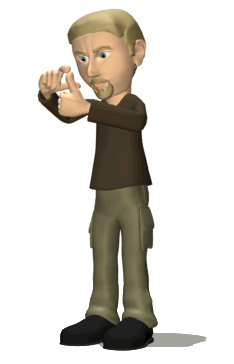Task Rank the acceleration  from least to greatest.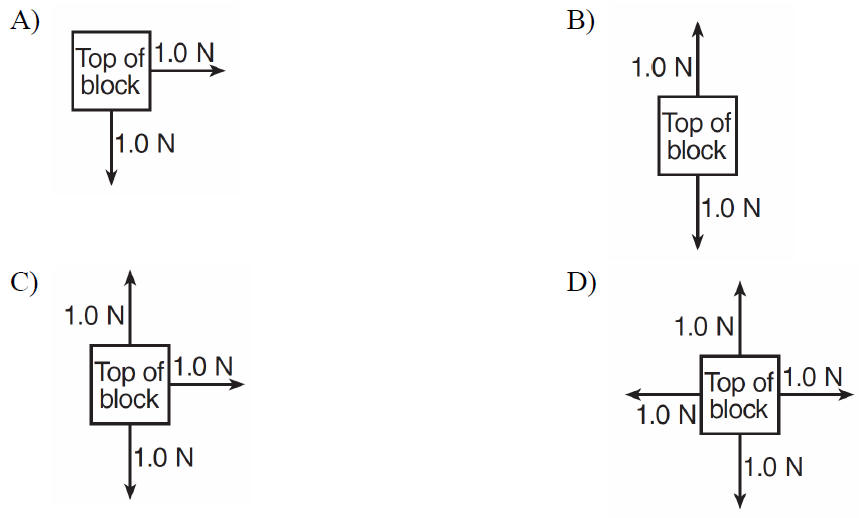Rank the accelerations from least to greatest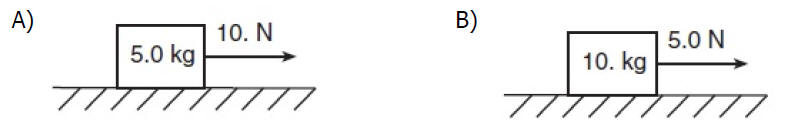3rd Law  Of Motion